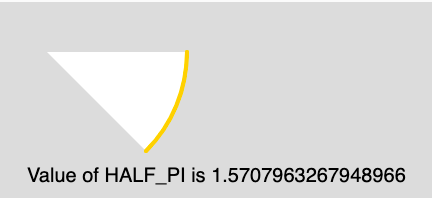Related Articles
p5.js | Constants | HALF_PI
• Last Updated : 12 Apr, 2019

The HALF_PI is a mathematical constant and its value is 1.57079632679489661923. The HALF_PI is the half ratio of the circumference of a circle to its diameter.

Syntax:

`HALF_PI`

Below program illustrates the usage of HALF_PI in p5.js:

Example:

 `function` `setup() { ` ` `  `    ``// Create Canvas of size 300*200 ` `    ``createCanvas(300, 200); ` `} ` ` `  `function` `draw() { ` `     `  `    ``// Set the background Color ` `    ``background(220); ` `     `  `    ``// Set the stroke color ` `    ``stroke(255, 204, 0); ` `     `  `    ``// Set the stroke weight ` `    ``strokeWeight(4); ` `     `  `    ``// Use of constant HALF_PI ` `    ``arc(50, 50, 280, 280, 0, HALF_PI/2);  ` `     `  `    ``noStroke(); ` `     `  `    ``// Set the font size ` `    ``textSize(20); ` `     `  `    ``// Display result ` `    ``text(``"Value of HALF_PI is "` `            ``+ HALF_PI, 30, 180); ` `} `

Output:Reference: https://p5js.org/reference/#/p5/HALF_PIMy Personal Notes arrow_drop_up
Recommended Articles
Page :